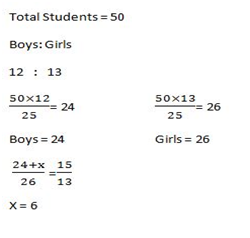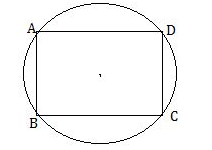# RRB ALP 2018 Practice Test Papers | Arithmetic Questions (Day-53)

Dear Aspirants, Here we have given the Important RRB ALP & Technicians Exam 2018 Practice Test Papers. Candidates those who are preparing for RRB ALP 2018 can practice these Arithmetic Questions to get more confidence to Crack RRB 2018 Examination.

[WpProQuiz 2709]

Click “Start Quiz” to attend these Questions and view Solutions

1. Simplify

2835÷9÷3

1. 110
2. 100
3. 105
4. 150
1. In a class there are 50 students. The ratio of Boys to Girls is 12:13. How many more boys would added so that the ratio is become 15:13?
1. 4
2. 6
3. 8
4. 10
1. A can do a piece of work in 4 days. B can do a same work in 6 days. With the help of C they can do finish the job in 2 days. Then how long C alone took to complete the work?
1. 10 days
2. 12 days
3. 15 days
4. 8 days
1. The difference of the Square of the two Consecutive Natural Numbers is 97. Find the large one
1. 49
2. 50
3. 48
4. 51
1. If a train, with a speed of 30 kmph crosses a pole in 60seconds. The length of the train is?
1. 1000m
2. 500 m
3. 750 m
4. 800 m
1. ABCD is a cyclic parallelogram. The Angle of∠ B is equal to
1. 45°
2. 60°
3. 30°
4. 90°
1. The difference between the length and breadth of a rectangle is 24m. If its perimeter is 100m, then its area is?
1. 144
2. 126
3. 156
4. 169
1. Which of the following fraction is Smallest?

10/13 , 16/26, 27/29, 32/51

1. 16/26
2. 27/29
3. 32/51
4. 10/13
1. The ratio of father’s age to his son’s age is 4 : 1. The product of their age is 324. What is the difference between the present ages of father and son?
1. 30
2. 25
3. 27
4. 28
1. What is the sum of all the natural numbers from 1 to 40?
1. 800
2. 820
3. 840
4. 860

2835 ×1/9 ×1/3 =105A = 1/4                  B = 1/6                  A+B = 5/12

A+B+C = 1/2

C = 1 /2 – 5 /12 = 6 /12 – 5/ 12 = 1/ 12

C alone can complete the job in 12 days

(x+1)2 – x2 = 97

X2  + 2x + 1 – x2  = 97

2x + 1 = 97

2x = 98

X = 48

X + 1 =49

Distance  = Speed × Time

30×(5/18) ×60 = 500 mABCD is a cyclic parallelogram

∴∠B+∠C = 180°

2∠B = 180°

∠B = 90°

L – B = 24 —–> (1)

Perimeter of Square = 2(L+B) = 100

(L+B) ——-> (2)

Solve (1) & (2)

L+B = 100

L – B = 24

2L = 26

L =13                   B = 12

Area = L×B = 13×12 = 156

16/26 = 0.615

27/29 = 0.931

32/51 = 0.627

10/13 = 0.769

4x ×x = 324

4x2 = 324           X2= 81                 X= 9

Father’s present age = 36            son’s Present age = 9

Difference of theirs Ages = 27

Sum of natural Numbers = n(n+1)/2

= 40(40+1)/2 = 40×41/2

= 41× 20 = 820

RRB ALP 2018 – “All in One” Study Materials and Practice Sets